# Quantum Phenomena

Photoelectric effect, electron energy levels, wave-particle duality

The Photoelectric Effect

·      Electrons are emitted from the surface of a metal when electromagnetic radiation above a certain frequency is shone on it.

-   There is no emission of electrons below the ‘threshhold frequency, fmin’ (which is different for every metal)

-   Above the threshold frequency, the numbers of electrons emitted is proportional to the intensity of radiation

-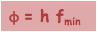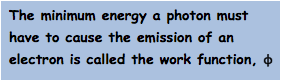Below the threshold frequency no electrons are emitted no matter how intense the radiation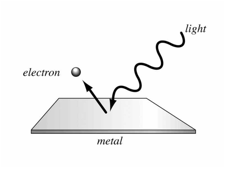·      Wave model of light cannot explain this effect as in this theory more intense waves always carry more energy

·      The photon theory of light is required, which describes light as small packets with energy proportional to their frequency – 1 ‘packet’ of energy is 1 quanta

·      An electron is emitted from the surface when one electron in the metal absorbs one photon of light with enough energy to cause it to leave the surface

·Any extra energy above this minimum gives the emitted photoelectron kinetic energy:

Electron Energy Levels in Atoms

·      One consequence of quantum theory is that electrons must occupy specific energy levels in atoms

·      The lowest energy level is called the ground state

·      Excitation is when an electron moves up to a higher energy level – it requires energy to do this from either absorbing a photon or a collision with a free elctron

·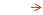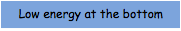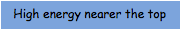De-excitation is when an electron moves down to a lower energy level – it emits energy as a photon of electromagnetic radiation

·Excitation can occur when a photon hits an atom but only when the energy of the photon is greater than or equal to the energy level jump of the electron

Fluorescent Tubes

·      Low density mercury vapour in a tube is ionized

·      Electrons in the mercury are excited as they collide with one another and with free electrons

·      The excited electrons then de-excite giving off photons with wavelengths in the UV part of the spectrum

·      These UV photons hit the inside surface of the glass tube that is coated with a fluorescent material

·      They are absorbed by electrons in the fluorescent material causing excitation

·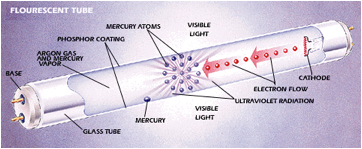These electrons then de-excite indirectly giving off photons of visible lightSpectra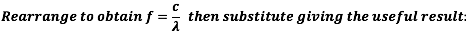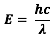Wave-Particle Duality

·      Normally we think of light as a wave – this helps to explain phenomena like diffraction

·      For effects like photo-electricity however this is not sufficient and it is useful to think of light as having a particle like nature which we call photons

·      This thinking also works in reverse. If we take an object like an electron it is usual to think of it as a particle but it also has a wave like nature. E.g. diffraction can be done with a beam of electrons

·      This dual nature of electromagnetic radiation and matter is called wave-particle duality

·      The wavelength of matter can calculated as the De-Broglie wavelength, _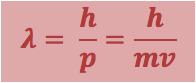_ = de Broglie wavelength (m)

h = Plancks constant = 6.63 x 10-34Js

p = momentum (kgms-1)

m = mass (kg)

v = velocity (ms-1)To do:

Describe  experiments to demonstrate the following:

-   The wave nature of light

-   The particle nature of light

-   The wave nature of electrons